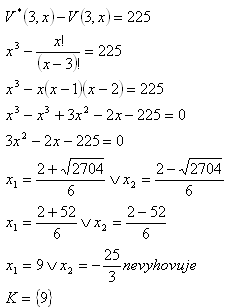sk|cz|

# Permutations (general)

1.Define and characterize permutations and permutations with repetition.

Solution:

Permutations

A permutation is an ordered sequence of k elements selected from a given finite set of n numbers, without repetitions, and not necessarily using all n elements of the given set.

Number of possible permutations: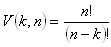Permutations with repetition

A permutation is an ordered sequence of k elements selected from a given finite set of n numbers, with repetitions, and not necessarily using all n elements of the given set.

Number of possible permutations with repetition:

V*(k,n) = nk

2. From a given set M = {a,b,c,d} enumerate the permutations with and without repetition for k=2. Determine their number.

Solution:

a) Permutations: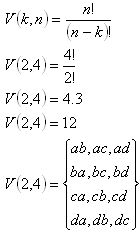b) Permutations with repetition: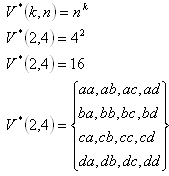3. There are 7 members in a committee. How many ways are there to choose a chairman, deputy chairman, secretary and a cash keeper?

Solution:
Permutations, n = 7, k = 4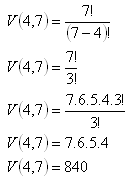There are 840 ways of choosing the four officials.

4.Eight students promissed to send a postcard each other. How many postcards did they send together?

Solution:
Permutations, n = 8, k = 2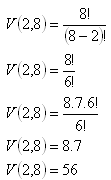The students sent 56 postcards.

5.From how many numbers 240 permutations can be made if the number of elements to be selected is 2?

Solution:
Permutations, n = x, k = 2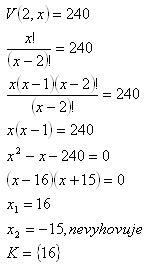16 numbers are needed.

6.If the number of members increments by 2, the number of possible variations with k=3 increments by 384. How many members are there?

Solution: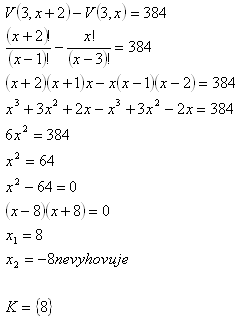There are 8 members.

7. You have 6 different tickets in your pocket marked with numbers 1-6. How many ways are there to choose 3 of them (considering the order), if
a) the selected ticket is not returned to the pocket.
b) the selected ticket is returned to the pocket.

Solution:
a)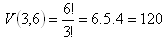b)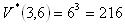The tickets can be selected in 120 or 216 ways.

8.Number of permutations without repetition with k=3 from x members is lower than number of permutations with repetition with k=3 from x members by 225. How many members are there?

Solution: